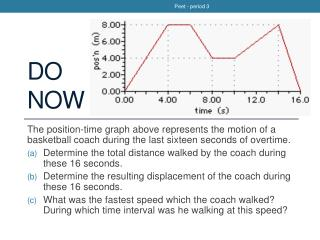Download PresentationDo Now

Loading in 2 Seconds...

# Do Now - PowerPoint PPT Presentation

Do Now. The position-time graph above represents the motion of a basketball coach during the last sixteen seconds of overtime. Determine the total distance walked by the coach during these 16 seconds. Determine the resulting displacement of the coach during these 16 seconds.I am the owner, or an agent authorized to act on behalf of the owner, of the copyrighted work described.
Download Presentation## Do Now

An Image/Link below is provided (as is) to download presentation

Download Policy: Content on the Website is provided to you AS IS for your information and personal use and may not be sold / licensed / shared on other websites without getting consent from its author.While downloading, if for some reason you are not able to download a presentation, the publisher may have deleted the file from their server.

- - - - - - - - - - - - - - - - - - - - - - - - - - E N D - - - - - - - - - - - - - - - - - - - - - - - - - -
Presentation Transcript
1. Peet - period 3 Do Now The position-time graph above represents the motion of a basketball coach during the last sixteen seconds of overtime. Determine the total distance walked by the coach during these 16 seconds. Determine the resulting displacement of the coach during these 16 seconds. What was the fastest speed which the coach walked? During which time interval was he walking at this speed?

2. Peet - period 3 Aim: How arevelocity vs. time graphs analyzed? SWBAT: construct and interpret graphs of position, velocity, or acceleration versus time (S4.5.1.i) determine and interpret slopes and areas of motion graphs (S4.5.1.ii)

3. Peet - period 3 Graphs of Motion * the steeper the slope, the higher the speed(value of velocity) • Position (displacement) vs. Time • Slope of position-time graph = velocity over that interval of time position position position position time time time time Slope is zero ∴velocity is zero (object at rest) Slope is positive ∴velocity is constant, positive Slope is negative ∴velocity is constant, negative Slope is curve ∴velocity is not constant (object accelerating)

4. Peet - period 3 Graphs of Motion * the steeper the slope, the higher the value of acceleration • Velocity vs. Time • Slope of v-t graph = acceleration over that interval of time velocity velocity velocity velocity time time time time Slope is zero ∴acceleration is zero (at rest or with constant velocity) Slope is positive ∴acceleration is constant, positive Slope is negative ∴acceleration is constant, negative Slope is curve ∴acceleration is not constant

5. Peet - period 3 • BEWARE: Just because the slope of the v-t graph is negative (acceleration negative), does not always mean the object in motion is decelerating (slowing down)! • Nor does the slope being positive mean the object is accelerating (speeding up) Slowing down Speeding up velocity time Slowing down Speeding up

6. Peet - period 3 Graphs of Motion * Sum of areas with signs: displacement * Sum of areas without signs: distance • Velocity vs. Time • Area under v-t graph = displacementand/or distance over that interval of time velocity velocity velocity time time time Line along x-axis No area ∴no displacement Line below x-axis Negative area Line above x-axis Positive area

7. Peet - period 3 B A C D

8. Peet - period 3 • What is the acceleration from: • t = 0s to t = 4s? • t = 4s to t = 8s? • t = 8s to t = 10s? What is the distance traveled? Is it the same as the object’s displacement?

9. Peet - period 3 position-time acceleration-time

10. Peet - period 3 Picture from Problem 3.82/3.87 Draw the p-t graph and a-t graph for this object’s motion.

11. Peet - period 3

12. Peet - period 3

13. Peet - period 3 Two Equations from Monday • Position with constant acceleration: • Velocity with constant acceleration:

14. Peet - period 3 Equations of Motion for Uniform (Constant) Acceleration

15. Peet - period 3 Example: 3.27 • A race car travels on a racetrack at 44 m/s and slows at a constant rate to a velocity of 22 m/s over 11 s. How far does it move during this time?

16. Peet - period 3 Problem 3.28 • A car accelerates at a constant rate from 15 m/s to 25 m/s while it travels a distance of 125 m/ How long does it take to achieve this speed?

17. Peet - period 3 Problem 3.29 • A bike rider pedals with constant acceleration to reach a velocity of 7.5 m/s over a time of 4.5 s. During the period of acceleration, the bike’s displacement is 19 m. What was the initial velocity of the bike?

18. Peet - period 3 Problem 3.30 • A man runs at a velocity of 4.5 m/s for 15 minutes. When going up an increasingly steep hill, he slows down at a constant rate of 0.05 m/s2 for 90.0 seconds and comes to a stop. How far did he run?